# DeepSinger: Singing Voice Synthesis with Data Mined From the Web

## Authors

* Equal contribution.

## Chinese

/ Sample 1 Sample 2 Sample 3
Data crawling
Lyrics: 爱从不容许人三心两意
Phonemes: PAD ai c ong b u r ong x v r en s an x in l iang PAD i

Lyrics: 遮住你的眼睛
Phonemes: zh e zh u n i d e PAD ian j ing

Lyrics: 好久好久
Phonemes: h ao j iou h ao j iou
Singing and accompaniment separation
Lyrics-to-singing alignment Attention map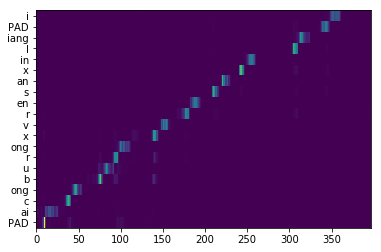Lyrics-to-singing alignment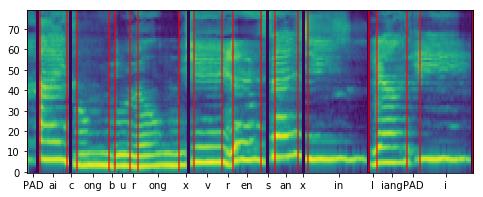Attention map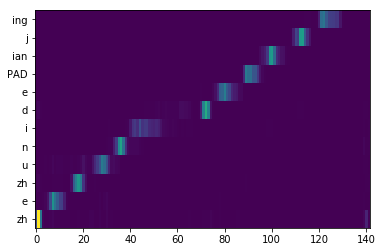Lyrics-to-singing alignment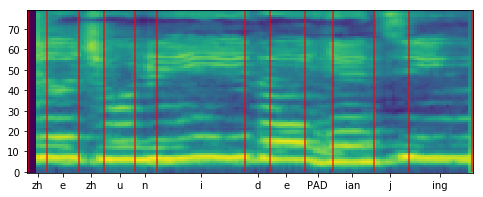Attention map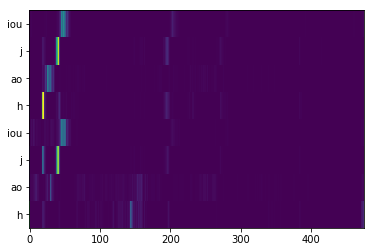Data filtration Keep (Splitting Reward: $\mathcal{O} = 0.8244$) Keep (Splitting Reward: $\mathcal{O} = 0.8359$) Discard (Splitting Reward: $\mathcal{O} = 0.3764$)
Singing modeling $\textit{GT (Linear+GL)}$

$\textit{DeepSinger}$

Pitch Plot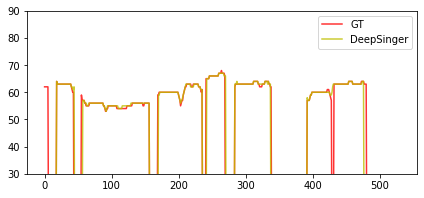$\textit{GT (Linear+GL)}$

$\textit{DeepSinger}$

Pitch Plot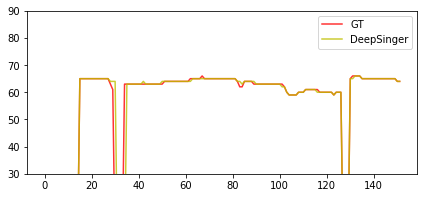/

P.S. 1) $\textit{GT}$, the ground-truth audio; 2) $\textit{GT (Linear+GL)}$, where we synthesize voices based on the ground-truth linear-spectrograms using Griffin-Lim; 3) $\textit{DeepSinger}$, where the audio is generated by DeepSinger.

## Cantonese

/ Sample 1 Sample 2 Sample 3
Data crawling
Lyrics: 我已经不懂心痛
Phonemes: ŋ o5 j i5 ɡ inɡ1 b a1 t d unɡ2 s a1 m t unɡɜ

Lyrics: 或你会了解在孤单中的心痛
Phonemes: w aa6 k n ei5 w ui6 l iu5 ɡ aai2 z oi6 ɡ u1 d aa1 n z unɡ1 d i1 k s a1 m t unɡɜ

Lyrics: 唔知点解 我成日都好担心
Phonemes: nɡ4 z i1 d i2 m ɡ aai2 ŋ o5 s inɡ4 j a6 t d ou1 h ou2 d aa1 m s a1 m
Singing and accompaniment separation
Lyrics-to-singing alignment Attention map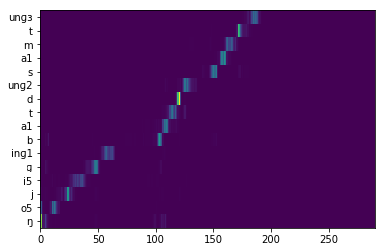Lyrics-to-singing alignment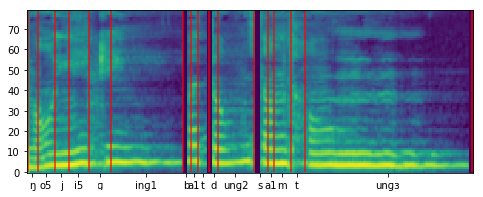Attention map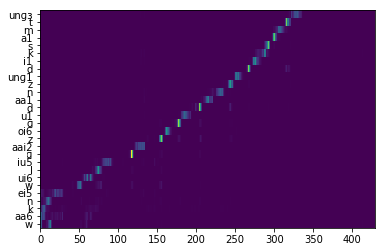Lyrics-to-singing alignmentAttention mapData filtration Keep (Splitting Reward: $\mathcal{O} = 0.8105$) Keep (Splitting Reward: $\mathcal{O} = 0.7372$) Discard (Splitting Reward: $\mathcal{O} = 0.3601$)
Singing modeling $\textit{GT (Linear+GL)}$

$\textit{DeepSinger}$

Pitch Plot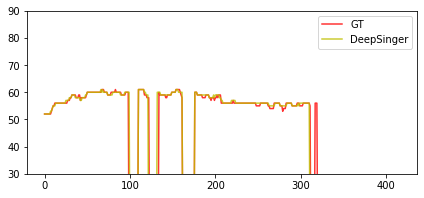$\textit{GT (Linear+GL)}$

$\textit{DeepSinger}$

Pitch Plot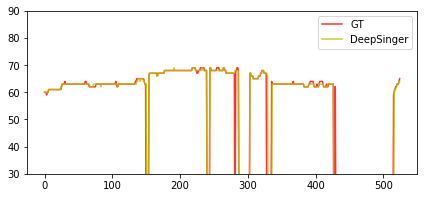/